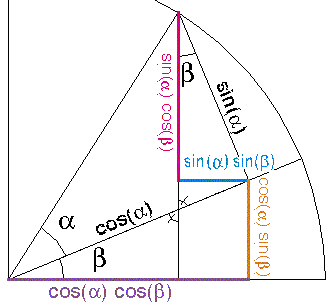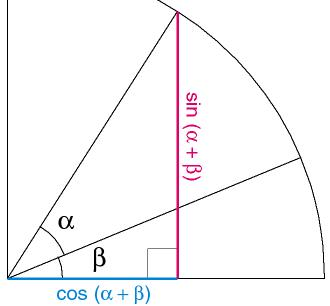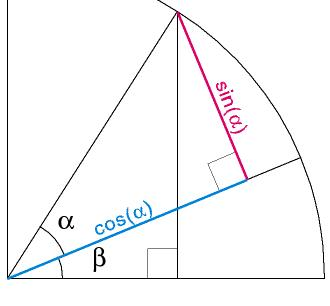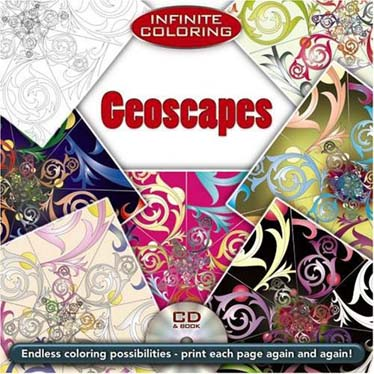First two frames use definition of cos and sin (circle is unit circle) 3rd frame: opposite angles are equal, Angles of a triangle add up to 180 therefore top angle of upper right triangle is Beta 4th frame: Erect a perpendicular off of sin(alpha) line - this makes a small right triangle whose right most angle is Beta (because this angle added to the angle opposite beta = 90. cos(a+b) = cos(a) cos(b) - sin(a) sin(b) and sin(a+b) = sin(a) cos(b) + sin (b) cos(a)

Here's the same demonstration in still pictures:Step 1:  Definition of sin and cosine Step 2:  Definition of sine and cosineStep 3:  Opposite angles are equal, triangle angles sum to 180 degrees Step 4: Erect a perpendicular from the vertical line. Step 5 (to the right): From step 4 see the upper tirangle whose hypotenuse  is sin(alpha) The side opposite angle beta is hypotenuse * sin(beta) or sin(alpha) * sin(beta) The leg adjacent to beta of the same triangle is hypotenuse * cos(beta) or sin(alpha) * cos(beta) - Then the triangle at the bottom with purple and orange legs has hypotenuse of cos(alpha). It's  legs are cos(alpha) * cos(beta)  (purple leg) and cos(alpha) * sin(beta)  (orange leg). - Adding the red and orange lines gives the vertical distance and subtracting the green from the purple line gives the horizontal distance.-
Hop's Other trigonometry Pages
-
Triangle angles add up to 180 degrees
What is sine and cosine?
Angle measure in radians, generating a sine wave
Sin(h)/h approaches 1 as h approaches zero
Pythagorean Theorem
-
 Hop David Coloring BooksA Hop David coloring book of Escher like tessellationsA Hop David coloring book of various geometrical landscapes. To be released in February of 2009. A lot of pages are devoted to space filling polyhedra with an emphasis on octahedra alternating with tetrahedra. There's also some polyhedra studies, logarithmic spirals, fractals (only the first few iterations or they'd be impossible to color!) and some miscellaneous.This book includes a CD to print out pages. I think these coloring books will be a good tool for teachers in helping get kids interested in math.
-
Hop's Gallery. Please check out my artwork!